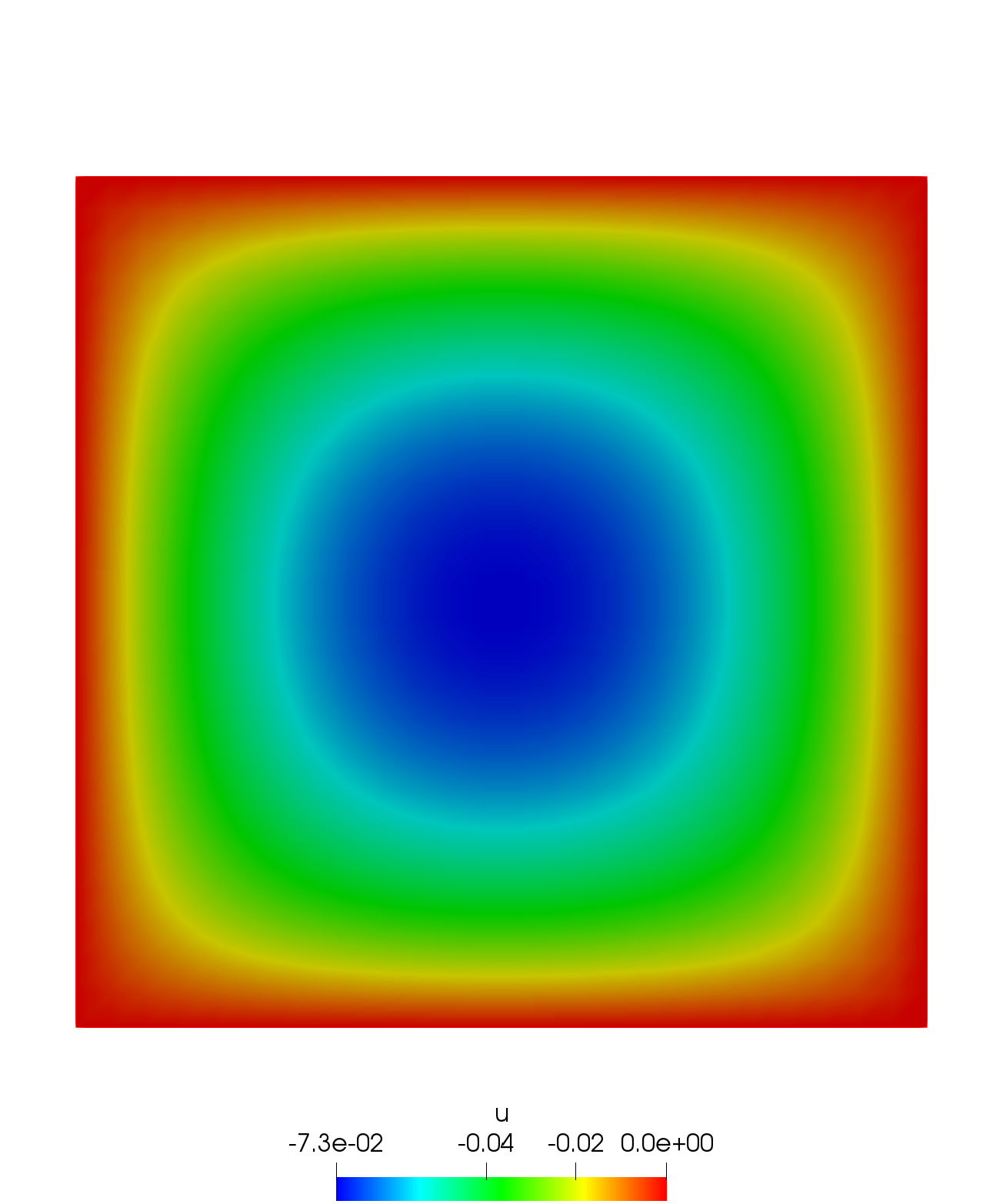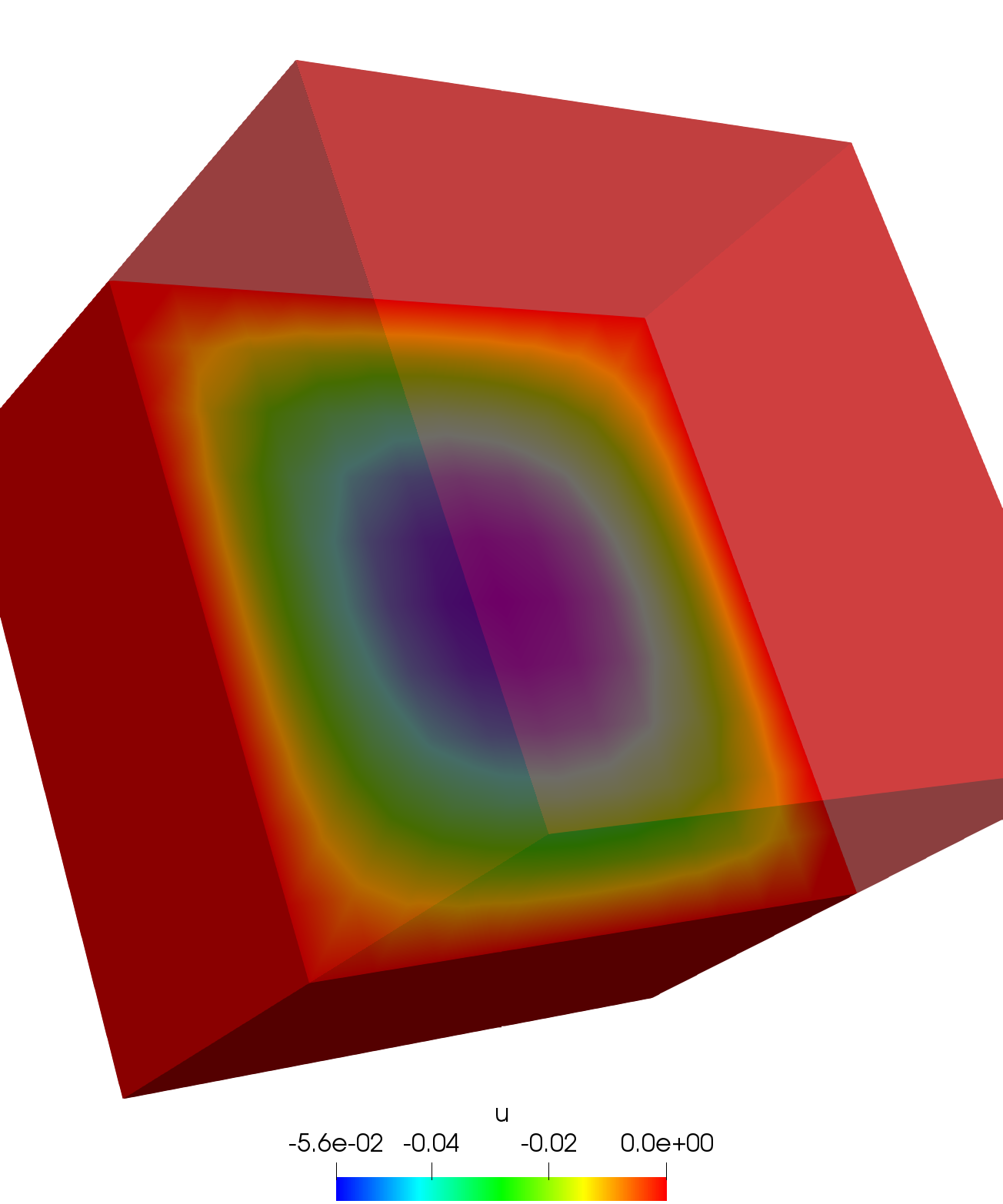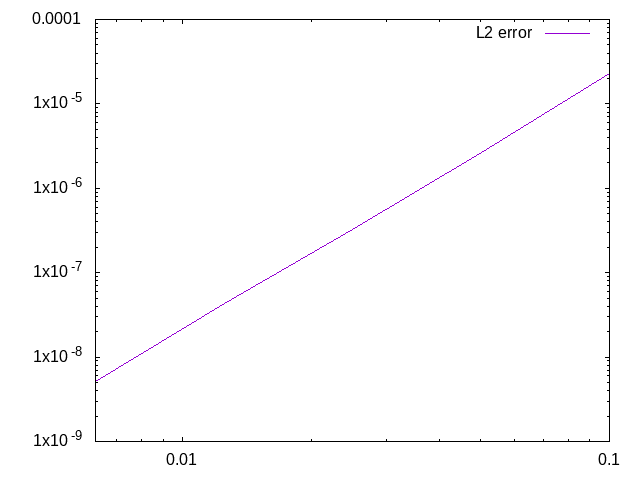Poisson

Algorithms for solving the 2D and 3D Poisson’s equation

Problem

Let $u\in\mathbb{R}$, solve:

$-\displaystyle{\Delta u = f}$

With $f$ an external force.

Variational form

Let $\Omega\in\mathbb{R}^n$, $2\leq n\leq3$. Let $u, v\in H^1(\Omega)$. The variational form reads as follows:

$\displaystyle{-\int_{\Omega}{\Delta u v} - \int_{\Omega}{f v} = 0}$

Using the Green formula:

$\displaystyle{\int_{\Omega}{\nabla u \cdot \nabla v} - \int_{\Omega}{f v} = 0}$

Algorithms

2D

Poisson’s equation on a square.

Result3D

Poisson’s equation on a cube.

ResultValidation

2D

Let $u_e$ be the analytical solution on $\Omega=[0,1]^2$:

$\displaystyle{ u_e = x(1-x)y(1-y)e^{x-y} }$

We get, for homogenous Dirichlet conditions on $\partial\Omega$:

$\displaystyle{ f = -2x(y-1)(y-2x+xy+2)e^{x-y} }$

and the following convergence curve:

Convergence curveThe slope obtained with this algorithm is equal to $3.0235$, which corresponds to the theoretical value of $3$.

The complete validation script is available here

Authors

Author: Simon Garnotel